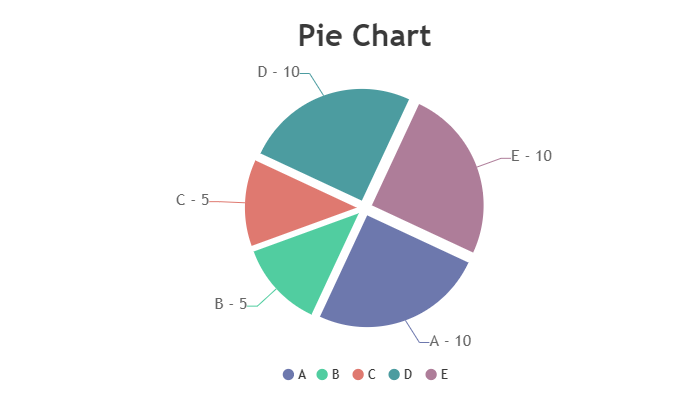Uh Oh! It seems you’re using an Ad blocker!

We always struggled to serve you with the best online calculations, thus, there's a humble request to either disable the AD blocker or go with premium plans to use the AD-Free version for calculators.

Or# Pie Chart Calculator

Enter the Group (variable) in the tool and the calculator will generate the pie chart for the values entered.

Group A :

Group B :

Group C :

Group D :

Table of Content

Get the Widget!

Add this calculator to your site and lets users to perform easy calculations.

Feedback

How easy was it to use our calculator? Did you face any problem, tell us!

The pie chart calculator determines the percentage and the degree of the angles of the statistical data. Just enter the values of the variables in the percentage chart calculator to identify all relative percentages and angles in degrees.

## Pie Chart:

A pie chart is a type of graph that is used to represent the data in a circular diagram. The wedges of the pie chart represent the pictorial representation of various variables.

Each variable has a certain portion of a certain pie chart percentage and is represented by a certain color in the 360-degree circle. The pie chart calculator needs a list of categorical variables and their numerical values to make a certain pie chart.

## Formula of the Pie Chart:

The formula for the pie chart with percentages of each variable is given by:

Percentage of variables  =  (Each variable /Total data values) × 100

Now for finding the angle of each variable in degrees:

Angle of variables  =  (Each variable /Total data values) ×  360°

## How to Create a Pie Chart?

Pie charts are the graphical representation of the data in which data is distributed. The pie chart provides an accurate and clear representation of the data.

Step 1:

Identify all the variables in the pie chart and find the relative count of each of the variables.

Step 2:

Sum all the variables in the pie chart to find the total sum of all the values of the variables.

Step 3:

Divide each value by the total ad multiplied with the 100, to find the percentage pie chart maker of each variable.

Step 4:

In the last step, you need to find how many degrees for each variable should be allocated. This can be done by multiplying with the 360 degrees.

## Practical Example:

Let’s suppose the favorite sport of each student in a class of 40.

Football 10, Hockey 5, Cricket 5, Basketball 10, and Badminton 10. Now represent the data in the form pie chart percentage of each student’s favorite sports and the relative angle.

### Solution:

A =  Football = 10

B = Hockey = 5

C = Cricket  = 5

#### Percentage of Variable:

The percentages of the variable of various games are:

A =  Football = (10/40) 100 = 25%

B = Hockey = (5/40) = 12.5 %

C = Cricket  = (5/40) = 12.5 %

D = Basketball = (10/40) 100 = 25%

E = Badminton =10/40) 100 = 25%

#### Angles of Variable:

The angles  of the variable of various games are:

A =  Football = (10/40) 360° = 90°

A =  Hockey= (5/40) 360° = 45°

A =  Cricket  = (5/40) 360° = 45°

A =  Basketball = (10/40) 360° = 90°

A =  Badminton = (10/40) 360° = 90°

#### Tabular Representation Variable:

The percentage and angle of the variable are given in the table below:

 Group Games Values Percentage Angle A Football 10 25 % 90° B Hockey 5 12.5 % 45° C Cricket 5 12.5 % 45° D Basketball 10 25 % 90° E Badminton 10 25 % 90°

#### Draw the Pie Chart:

The favorite game of the students the percentage pie chart and the angles of each game are given in the chart below:You can calculate the relative percentage of each game and their angles with the percentage pie chart calculator. The pie chart makes it possible to understand the percentage of each variable from the total percentage.

## What Pie Chart Calculator Calculates?

The pie chart percentage maker reveals the following data for the users:

#### Pie Chart Percentage Variable:

The pie chart percentage of each variable from the total of all the values.

#### Pie Chart Angles of Variable:

The relative angle of each variable is calculated by multiplying it by 360 degrees.

The pie chart percentage calculator calculates the pictorial representation of the data variable and their percentage along with the relative angle.

## FAQs:

### What Is the Other Name of the Pie Chart?

The other name of the pie chart is a circle chart. It is a way of summarizing a set of nominal data or displaying the different values of a given variable in the form of a pie chart with percentages.

### What Is a Pie Chart and a Column Chart?

Column chart: It is used for comparing data across categories.

Pie Chart: fUsed to show the relative shares of categories in a total

### What Are Pie Charts Forms?

The pie charts are divided into two forms,

• 2D pie chart
• 3D pie chart

The pie chart maker percentages provide 2D pie charts to show the relative share of the data values.

## References:

From the source of Wikipedia: Pie chart

From the source of chartio.com: What is pie Chart?